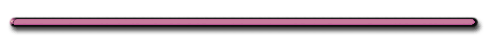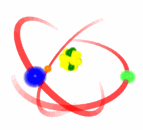HomeII. Explaining photoelectric effect using quantum theory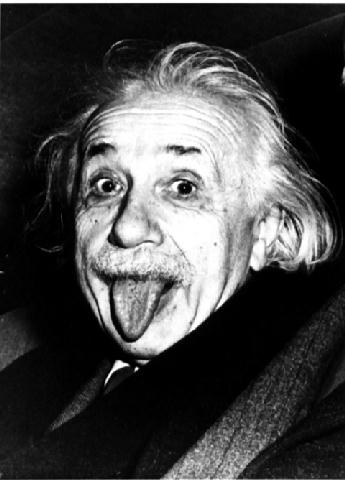A. Quantum Theory

1) electrons can only absorb or emit energy in discrete amounts called quanta (packets)

2) Energy of each quanta (photon) is proportional to frequency of radiation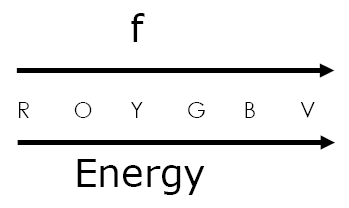Energy of a Quanta

 E = hf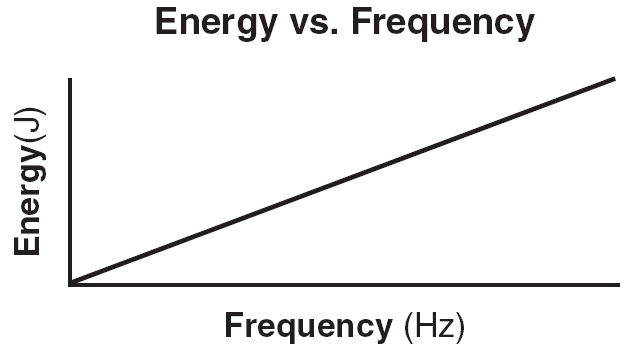June 2004 #40Max Planck h = Planck's constant  =6.63 X 10-34 Js

Since: c = fλ

c = 3 x 108 m/s

And f = c/λ

 E = hc/λEinstein, Photoelectric Effect